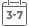# Real Analysis: A Constructive Approach

• Publish Date: 2006-11-28
• Binding: Hardcover
• Author: Mark Bridger
• Sale
• \$21.38
• Regular price \$190.16A unique approach to analysis that lets you apply mathematics across a range of subjects

This innovative text sets forth a thoroughly rigorous modern account of the theoretical underpinnings of calculus: continuity, differentiability, and convergence. Using a constructive approach, every proof of every result is direct and ultimately computationally verifiable. In particular, existence is never established by showing that the assumption of non-existence leads to a contradiction. The ultimate consequence of this method is that it makes sensenot just to math majors but also to students from all branches of the sciences.

The text begins with a construction of the real numbers beginning with the rationals, using interval arithmetic. This introduces readers to the reasoning and proof-writing skills necessary for doing and communicating mathematics, and it sets the foundation for the rest of the text, which includes:

• Early use of the Completeness Theorem to prove a helpful Inverse Function Theorem
• Sequences, limits and series, and the careful derivation of formulas and estimates for important functions
• Emphasis on uniform continuity and its consequences, such as boundedness and the extension of uniformly continuous functions from dense subsets
• Construction of the Riemann integral for functions uniformly continuous on an interval, and its extension to improper integrals
• Differentiation, emphasizing the derivative as a function rather than a pointwise limit
• Properties of sequences and series of continuous and differentiable functions
• Fourier series and an introduction to more advanced ideas in functional analysis

Examples throughout the text demonstrate the application of new concepts. Readers can test their own skills with problems and projects ranging in difficulty from basic to challenging.

This book is designed mainly for an undergraduate course, and the author understands that many readers will not go on to more advanced pure mathematics. He therefore emphasizes an approach to mathematical analysis that can be applied across a range of subjects in engineering and the sciences.

MORE FROM THIS COLLECTION